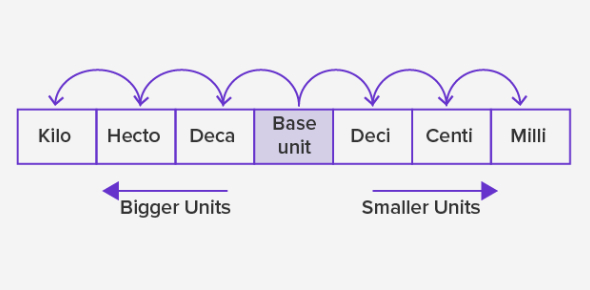# System Of Measurement Quiz: Metric System

20 Questions | Attempts: 8437SettingsThis is a system of measurement quiz about the metric system. Units can be described in many forms, and this is why there is a metric system. Have you been having a difficult time trying to understand the metric system? If so, then the quiz below is exactly what you need to help you study for the metric system test that is coming up. Give it a shot and be sure to countercheck with your notes. All the best!

• 1.
What is the unit of measure for mass?
• A.

Pounds

• B.

Liters

• C.

Grams

• D.

Meters

• 2.
Convert the following measurement: 1000 cm = _______ m
• A.

1

• B.

10

• C.

100

• D.

.001

• 3.
What is the most appropriate measurement of the amount of liquid in Starpoint's pool?
• A.

ML

• B.

KL

• C.

CL

• D.

Cg

• 4.
Which would have more density, a piece of wood with a mass of 250 grams or a piece of the SAME TYPE of wood with a mass of 1500 grams?
• A.

250 grams

• B.

1500 grams

• C.

Both are the same

• D.

Cannot tell from the information

• 5.
How many mL are there in 1 L?
• A.

1

• B.

100

• C.

1000

• D.

.001

• 6.
Volume can be expressed in what unit of measure?
• A.

Grams

• B.

Meters

• C.

Centimeters

• D.

Centimeters cubed

• 7.
A wall measures 3 meters long, 4 meter wide, and 2 meters high.  What is the area of the wall?
• A.

24 meters squared

• B.

12 meters squared

• C.

9 meters squared

• D.

Cannot tell from this information

• 8.
What tool is used to find mass?
• A.

Scale

• B.

Meter Stick

• C.

• D.

Balance

• 9.
To find the volume of an oddly shaped object, what scientific principle is used?
• A.

Measuring length, times width, times height

• B.

Finding the mass

• C.

Using displacement

• D.

Guessing

• 10.
The metric system is based on what number?
• A.

10

• B.

100

• C.

1

• D.

247

• 11.
Why do ice cubes float in a glass of water?
• A.

The ice weighs less than the water.

• B.

The ice weighs the same as the water.

• C.

The ice is less dense than the water.

• D.

The water is less dense than the ice

• 12.
What is NOT a unit of measure for distance?
• A.

Dkm

• B.

Cm

• C.

Mg

• D.

Mm

• 13.
In order to calculate the density of an object, a scientist must find:
• A.

Volume and area

• B.

Volume and mass

• C.

Mass and distance

• D.

Mass and area

• 14.
A dip in the water level inside of a graduated cylinder is called a:
• A.

Air bubble

• B.

• C.

Meniscus

• D.

Volume

• 15.
Which of the following statements are an observation?
• A.

Mr. Scully's tests are hard because everyone fails.

• B.

Starpoint Middle school says the pledge to the flag everyday.

• C.

The Mona Lisa is the best painting ever created.

• D.

The Buffalo Bills are the best football team in the NFL.

• 16.
When first entering a science room, what should you do with the equipment that is found on your table?
• A.

Whatever you want.

• B.

Carefully move the materials in front of you for better work conditions.

• C.

Do not touch it until you are told to do so.

• D.

Read the directions and immediately get to work.

• 17.
'Geese fly south for the winter because they do not like the cold.'  This statement is an example of:
• A.

An observation

• B.

An Inference

• C.

A scientific Method

• D.

A hypothesis.

• 18.
You think that putting the new 'Radial X2000' tires on your Mustang will make it accelerate faster.  Your hypothesis for this experiment would look like which of the following statements?
• A.

I think that putting Radial X2000 tires on my car will make it accelerate faster.

• B.

If I put Radial X2000 tires on my car it has to go faster than it did.

• C.

If I put Radial X2000 tires on my car, then it will go faster because they have better traction.

• D.

If I put new tires on my car then it will go faster than it did before.

• 19.
The last step in the Scientific Method says that you need to repeat the process over and over again.  Why would you do this?
• A.

Because as a scientist, you like doing experiments.

• B.

To test your results and conclude that you will get the same results everytime.

• C.

You really don't have to do the experiment over again, but its a good idea.

• D.

To test a new variable.

• 20.
Identify the qualitative observation.
• A.

The pizza tastes bad because it was left in the oven too long.

• B.

The pizza is expensive.

• C.

This pizza is the best in Niagara county.

• D.

The pizza has cheese and pepperoni on top.

## Related TopicsBack to top
×

Wait!
Here's an interesting quiz for you.